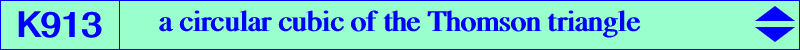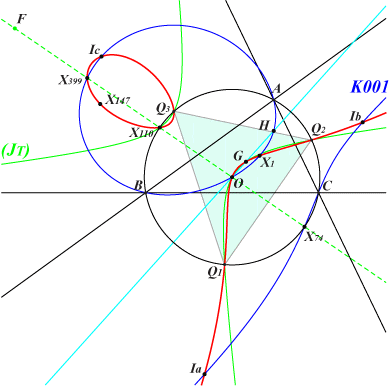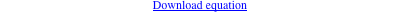too complicated to be written here. Click on the link to download a text file.X(1), X(2), X(3), X(30), X(110), X(147), X(399), X(5536) excenters vertices of the Thomson triangleK913 is a member of the pencil generated by two decomposed cubics : one is the union of the Euler line and the circumcircle, the other is the union of the line at infinity and the Thomson-Jerabek hyperbola. This pencil is stable under isogonal conjugation with respect to the Thomson triangle. It also contains K463, K834 which are the only self-isogonal cubics. See also K1095. All these circular cubics have their focus on the line X(3), X(74), X(110), etc. That of K913 is F, the homothetic of X(110) under h(X3, 3). F is now X(14094) in ETC (2017-08-07). Note that the foci of a cubic and its isogonal transform are inverse in the circumcircle of ABC. This pencil is actually the pencil K001, K187, etc, for the Thomson triangle. See K187 for further details. K913 is a member of another pencil generated by K001 and the union of the line at infinity and the Stammler hyperbola. See K800, a member of the pencil.